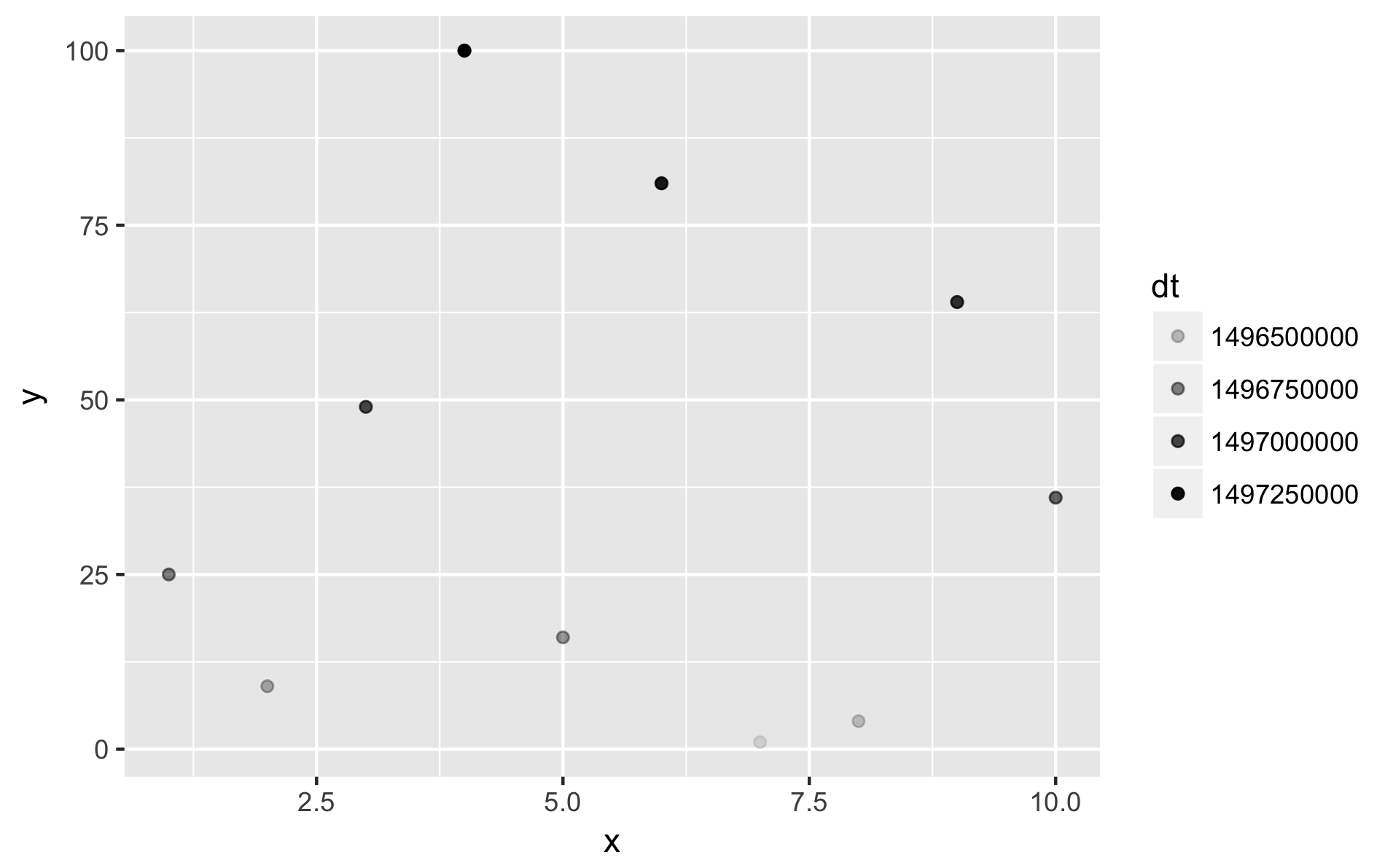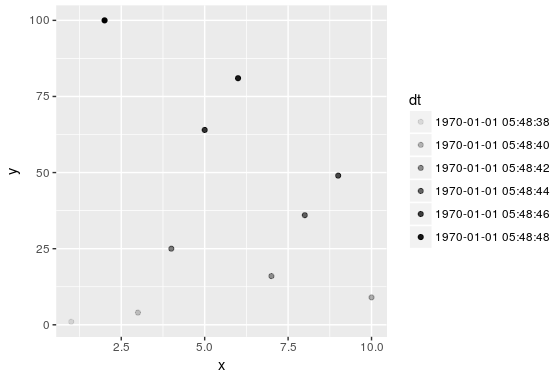{{ message }}

# rescale() works with Date, POSIXct and POSIXlt objects.#75

Merged
merged 2 commits into from Jun 26, 2017
Merged

# rescale() works with Date, POSIXct and POSIXlt objects.#75

merged 2 commits into from Jun 26, 2017

## Conversation

This file contains bidirectional Unicode text that may be interpreted or compiled differently than what appears below. To review, open the file in an editor that reveals hidden Unicode characters. Learn more about bidirectional Unicode characters

###zeehio commented Mar 1, 2016

 This pull request deals with #74 as discussed. Changes: documents the change in `NEWS.md` adds tests for rescaling dates (simple tests but enough to show and test the feature). edits `R/bounds.r`, checking for `Date`, `POSIXct` and `POSIXlt` inputs; converting them to numeric. Thanks for your time. Feel free to tell me if there is anything that does not convince you. The text was updated successfully, but these errors were encountered:

## Current coverage is 61.71% (diff: 100%)

Merging #75 into master will increase coverage by 0.57%

```@@             master        #75   diff @@
==========================================
Files            26         26
Lines           803        815    +12
Methods           0          0
Messages          0          0
Branches          0          0
==========================================
+ Hits            491        503    +12
Misses          312        312
Partials          0          0          ```

R/bounds.r Outdated
 #' If needed, converts input from classes that lack division and multiplication #' to classes that can be scaled. #' @param x vector of values to convert, if needed adapt_input_class <- function(x) {

###hadley Nov 1, 2016

This shouldn't be documented

###zeehio Nov 1, 2016

I left the comments as conventional R comments, so it does not appear under man/. I can remove the comments completely if you prefer.

R/bounds.r Outdated
 #' to classes that can be scaled. #' @param x vector of values to convert, if needed adapt_input_class <- function(x) { if (any(class(x) %in% c("Date", "POSIXct", "POSIXlt", "POSIXt"))) {

###hadley Nov 1, 2016

Please use `inherits()` here.

A better formulation would be:

```if (...) {
...
} else {
...
}```

as then you no longer need explicit `return()` statements..

###zeehio Nov 1, 2016

Done, thank you for your quick review.

###karawoo commented Jun 23, 2017

 This would be useful for some of the scales stuff I'm working on for ggplot2 – it would allow scaling alpha with datetimes, for one. But I think we need a way to go back to datetimes after the conversion, otherwise you get legends that look like this: ```dat <- data.frame( x = sample(1:10, 10), y = (1:10)^2, dt = seq( as.POSIXct("2017-06-01 15:28:44 PDT"), as.POSIXct("2017-06-12 15:28:44 PDT"), length.out = 10 ) ) ggplot(dat, aes(x, y)) + geom_point(aes(alpha = dt))`````` rescale() works with Date, POSIXct, POSIXt and POSIXlt objects. ```
``` 5e8ef20 ```

###zeehio commented Jun 23, 2017 • edited Loading

 @karawoo Thanks for reviewing this. I believe that what you are asking for fits better in the `ggplot2` package and not in the scales package. Here is your example, with a draft of what the `ggplot2::scale_alpha_datetime` could be: ``````library(ggplot2) dat <- data.frame( x = sample(1:10, 10), y = (1:10)^2, dt = seq( as.POSIXct("2017-06-01 15:28:44 PDT"), as.POSIXct("2017-06-12 15:28:44 PDT"), length.out = 10 ) ) scale_alpha_datetime <- function(name = waiver(), origin = "1970-01-01 00:00.00 UTC", ...) { origin <- force(origin) label_fun <- function(breaks) { as.POSIXct(breaks, origin = origin) } scale_alpha_continuous(name = name, labels = label_fun, ...) } ggplot(dat, aes(x, y)) + geom_point(aes(alpha = dt)) + scale_alpha_datetime() ``````The actual `scale_alpha_datetime` function should be closer to the other `scale_*_datetime` functions, to provide better label formatting and a more reasonable choice of date breaks. Link to the relevant ggplot2 issue: tidyverse/ggplot2#1526

R/bounds.r Outdated
 # If needed, converts input from classes that lack division and multiplication # to classes that can be scaled. # @param x vector of values to convert, if needed adapt_input_class <- function(x) {

###hadley Jun 23, 2017

I'm not sure I follow the reasoning here. Why not just unconditionally coerce to numeric?

###zeehio Jun 23, 2017

`scales::rescale` mainly depends on elementary arithmetic operations (addition, subtraction, multiplication and division). Coercing to numeric is a good way to define multiplication and division operations for `POSIXct` objects, but it is not the way to go for all R objects. For instance `integer64` numbers, from the `bit64` package, define their own arithmetic operators to prevent loss of precision.

If the coercion to numeric is done, there will be a warning when using some integer64 objects:

``````x <- bit64::as.integer64(c(2^50, 2^54+1, 2^60+2))
from <- range(x, na.rm = TRUE)
# I need to compute the `from` range here due to an unrelated bit64 bug triggered
# when range(..., finite = TRUE) is used. (this bug has already been
# reported to the bit64 maintainer)

# No warning given now:
scales::rescale(x = x, from = from)
#  0.00000000 0.01466276 1.00000000

# If we force coercion to numeric there is a warning:
scales::rescale(x = as.numeric(x), from = as.numeric(from))
# Warning messages:
# 1: In as.double.integer64(from) :
#   integer precision lost while converting to double
# 2: In as.double.integer64(x) :
#   integer precision lost while converting to double
#  0.00000000 0.01466276 1.00000000
``````

I wanted to provide an example were the `as.numeric` approach is wrong, but `scales::rescale` calls `scales::zero_range` that has a default tolerance too large for `integer64` data. I could work towards letting the user give a custom tolerance value, but for the sake of the explanation you can set `tol` to zero manually in the `zero_range` definition in `r/bounds.r` and rebuild, in case you want to reproduce this example:

``````x <- bit64::as.integer64(2^60) + 1:3
# x has three consecutive numbers, we expect the result to be c(0, 0.5, 1)
x_range <- range(x, na.rm = TRUE)
scales::rescale(x = x, from = x_range) # Correct result
#  0.0 0.5 1.0
scales::rescale(x = as.numeric(x), from = as.numeric(x_range)) # loss of precision
#  0.5 0.5 0.5
``````

Even if we have tolerance issues in this case, I prefer to work towards making `scales` work with other objects, and therefore not coercing everything to `numeric`.

But you have the last word, though 😃

###hadley Jun 23, 2017

In that case I'd prefer to use an S3 generic.

###zeehio Jun 26, 2017

Using an S3 generic is the best approach, my apologies for not thinking about them when I wrote this patch. I was now able to provide a method (and test case) for `integer64` data as well.

``` rescale and rescale_mid as S3 generics ```
``` 497a1f0 ```
`Provide support for Date, POSIXct, POSIXlt and integer64 types, as well as numeric.`

###hadley commented Jun 26, 2017

 Thanks!

added a commit to zeehio/scales that referenced this issue Oct 17, 2017
``` Provides a default method for rescale and rescale_mid ```
``` 224db0e ```
```The idea behind r-lib#74 and r-lib#75 was to use an S3 method so we never coerced to numeric
unless we had to. The aim was to prevent future cases similar to the integer64 one
where the coercion to numeric implies a loss of precision.

Reality is that there are many more numeric types out there that do not inherit from the numeric
class (like the dist object mentioned in r-lib#102), compared to the number of cases similar to integer64, where the numeric coercion is wrong.

- Not providing the `default` methods has the advantage that we only rescale the classes that we know can be rescaled, and the disadvantage that we get issues like r-lib#102 when we find a new object. This is what was implemented in r-lib#75

- Providing `rescale.default` and `rescale_mid.default` has the advantage of catching all the numeric-like types that do not inherit the numeric class (such as the dist object) and working as expected. However, we risk to deliver wrong results without an error if a case similar to integer64 appeared. This is what this commit implements.```
added a commit to zeehio/scales that referenced this issue Jun 9, 2018
``` Provides a default method for rescale and rescale_mid ```
``` cfabfae ```
```The idea behind r-lib#74 and r-lib#75 was to use an S3 method so we never coerced to numeric
unless we had to. The aim was to prevent future cases similar to the integer64 one
where the coercion to numeric implies a loss of precision.

Reality is that there are many more numeric types out there that do not inherit from the numeric
class (like the dist object mentioned in r-lib#102), compared to the number of cases similar to integer64, where the numeric coercion is wrong.

- Not providing the `default` methods has the advantage that we only rescale the classes that we know can be rescaled, and the disadvantage that we get issues like r-lib#102 when we find a new object. This is what was implemented in r-lib#75

- Providing `rescale.default` and `rescale_mid.default` has the advantage of catching all the numeric-like types that do not inherit the numeric class (such as the dist object) and working as expected. However, we risk to deliver wrong results without an error if a case similar to integer64 appeared. This is what this commit implements.```